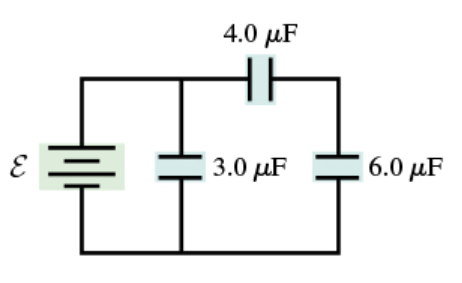# Problem: Consider the circuit shown in (Figure 1). Assume E = 16 V. What is the charge on 3.0 μF capacitor? Express your answer with the appropriate units.

###### FREE Expert Solution

The charge stored on a capacitor:

$\overline{){\mathbf{Q}}{\mathbf{=}}{\mathbf{C}}{\mathbf{V}}}$

The voltage across the 3.0 μF capacitor is 16 V.

C = 3.0 μF (10-6F/1μF) = 3.0 × 10-6 F

89% (42 ratings)###### Problem Details

Consider the circuit shown in (Figure 1). Assume E = 16 V. What is the charge on 3.0 μF capacitor? Express your answer with the appropriate units.